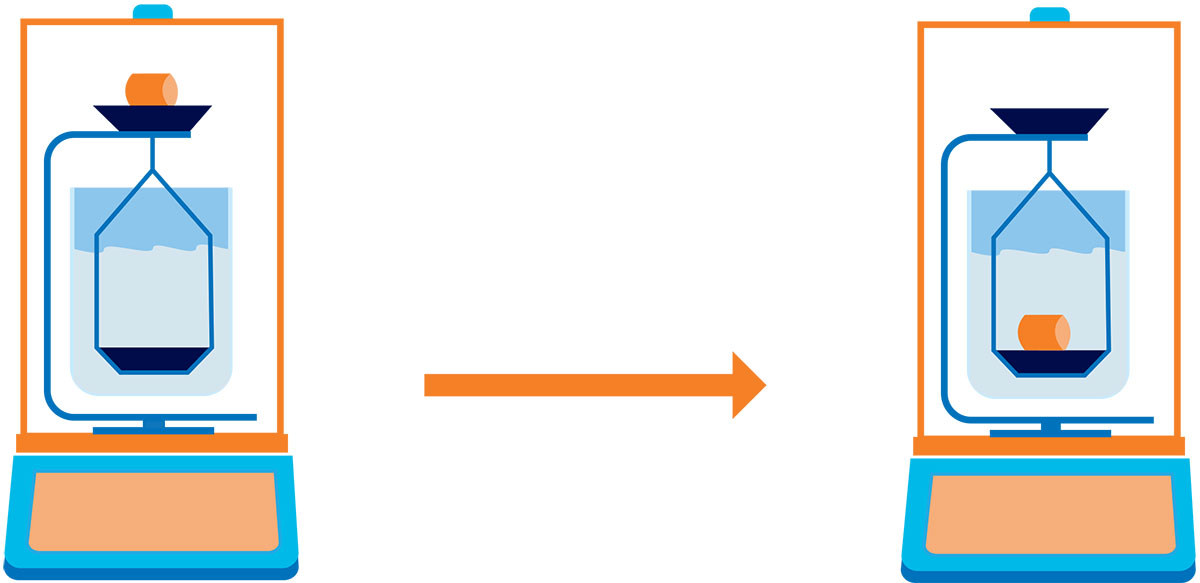## Density Determination of Solids and Liquids

The density of a sample is an important quality parameter of both raw materials and finished products. Various techniques enable the density of solid and liquid materials to be accurately determined. EAG Laboratories performs density analysis of solids and liquids using the gravimetric buoyancy method and the gas displacement method.

## Gravimetric buoyancy method

The most widely used method for determining density of solids and liquids is the gravimetric buoyancy technique which utilizes Archimedes’ principle. Archimedes’ principle states that a body immersed in fluid experiences a buoyant force acting upwards on it. The magnitude of this force is equivalent to the weight of the fluid displaced by the body.## Measuring principle for a solid sample

The sample is weighed once in air and once immersed in the auxiliary liquid with known density. The density of the solid sample can be determined from the known density of the liquid and the two mass values.

## Measuring principle for a liquid sample

A reference body with known volume (glass sinker) is weighed once in air and once in the liquid with unknown density (the sample). The density of the liquid can be determined from the known volume of the reference body and the two mass values.

## Gas displacement method

Gas pycnometry is a fast and efficient analysis technique to determine the skeletal density* of solid material samples, whether powdered or in one piece. This technique is based on the displacement of a volume of gas (mostly helium) by the solid space.

*Skeletal density: the ratio between sample mass and volume of the sample including the volume of closed pores (if present).

## Measuring principle

A sample is weighed and placed in a calibrated sample chamber of known volume. Helium is first loaded at a known pressure in a calibrated reference chamber and then expanded into the sample chamber. The equilibrated pressures for both steps are recorded by the instrument and the material volume is determined. The skeletal density is calculated from the determined material volume and the sample mass.

## Ideal Uses

• Density determination of solids (including powders) and liquids

## Strengths

Gravimetric buoyancy method – solid samples
• Short measurement times
• Flexible with regard to sample size
• Non-destructive method

Gravimetric buoyancy method – liquid samples
• Short measurement times
• Non-destructive method

Gas displacement method
• Suitable for solid samples, powders included
• Utmost precision in temperature control and pressure stabilization resulting in highly accurate and reproducible results
• Non-destructive method

## Limitations

Gravimetric buoyancy method – solid samples
• Not suitable for powders
• Samples must be free of open pores
• Air bubbles must not be trapped

Gravimetric buoyancy method – liquid samples<
• Air bubbles must not be trapped
• Measurement can only be performed at lab room temperature

Gas displacement method
• Relatively large amounts of sample required (30, 45, 65 or 110 cm3)
• Sample must fit in cylindrical sample chamber

## Technical Specifications

Gravimetric buoyancy method – solid samples
• Preferred minimum sample weight: 1 gram
• Accuracy: 3 digits after the decimal point

Gravimetric buoyancy method – liquid samples
• Minimum sample volume: 40 ml
• Accuracy: 3 digits after the decimal point

Gas displacement method
• Sample capacity: 30, 45, 65 cm3. Best analytical results will be obtained when the sample completely fills the sample chamber. The sample chamber must be filled for at least 66%.
• Accuracy: 2 to 3 digits after the decimal point